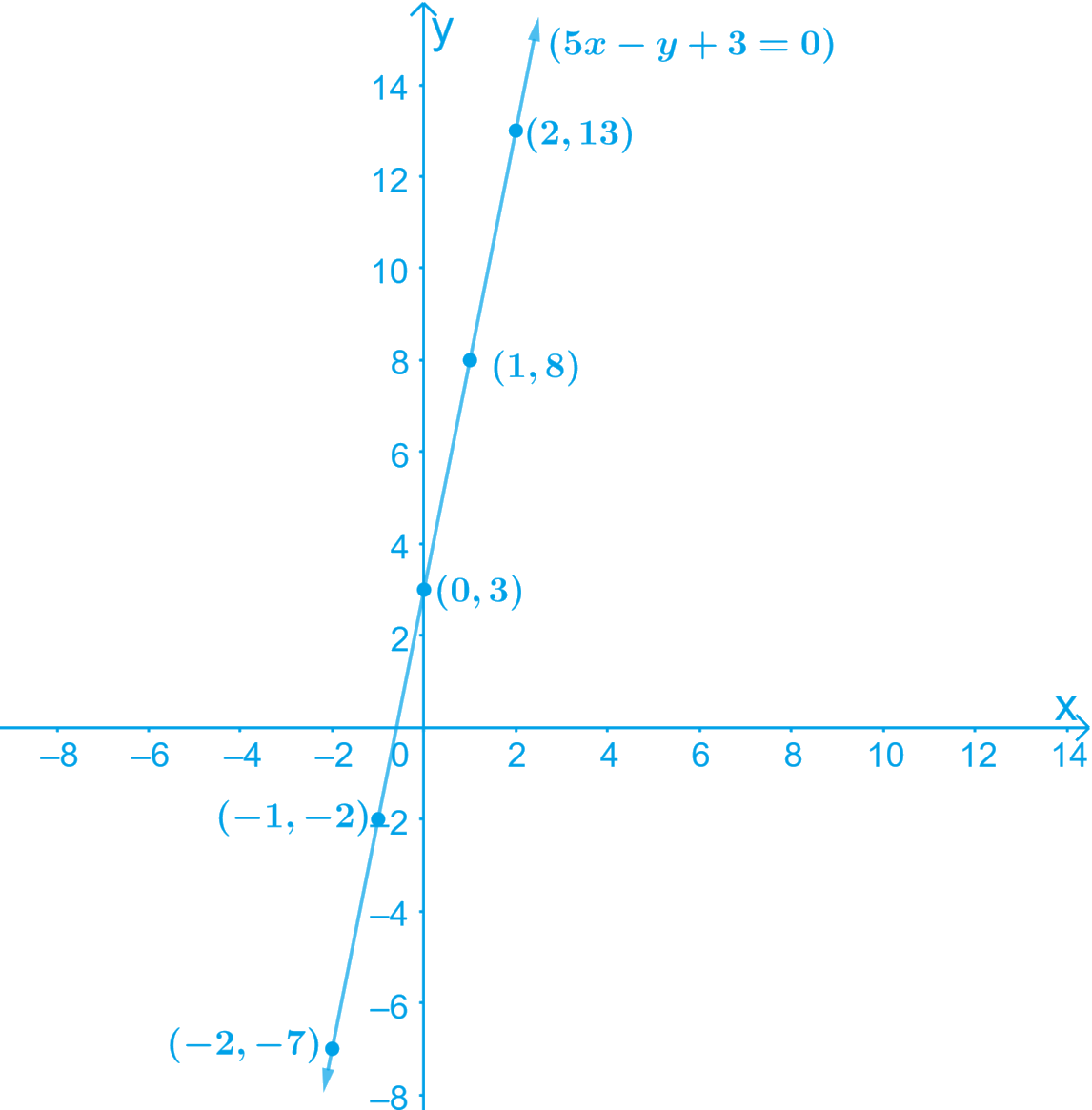# Ex.4.3 Q4 Linear Equations in Two Variables Solution - NCERT Maths Class 9

Go back to  'Ex.4.3'

## Question

The taxi fare in a city is as follows: For the first kilometer, the fare is $$₹\, 8$$ and for the subsequent distance it is $$₹\, 5 \,\rm{per \,km.}$$ Taking the distance covered as $$x$$ $$\rm{km}$$ and total fare as $$₹$$ $$y$$, write a linear equation for this information, and draw its graph.

Video Solution
Linear Equations In Two Variables
Ex 4.3 | Question 4

## Text Solution

What is known?

Distance covered is $$x$$ $$\rm{km}$$ and total fare is $$₹$$. $$y$$. Also fare of first kilometer is $$₹\, 8$$ and for subsequent distance is $$₹\, 5$$

What is Unknown?

Linear equation for given condition and also graph of it.

Reasoning:

We know fare for first $$\rm{km}$$ and add the fare of subsequent distance and the result will form linear equation. After that by finding the solution for linear equation we can draw graph of it.

#### Steps:

Given,

Let the Total distance covered $$= x\,\rm{km}$$

Let the Total fare covered $$= ₹$$ $$y$$

Therefore, subsequent distance $$= (x -1) \rm{km}$$

Fare for the subsequent distance $$= ₹ 5 (x − 1)$$

Fare for $$1$$st kilometer $$= ₹\, 8$$

The linear equation for the above information is given by,

Total fare

\begin{align} &(y) = [ 8 + ( x - 1 ) 5 ] \\ &y = 8 + 5 x - 5 \\ &y = 5x + 3\\ &5 x - y + 3 = 0 \end{align}

Re-write the equation by transposing as $$y = 5x + 3 \qquad \dots \dots \text{ Equation } (1)$$

By substituting different values of $$x$$ in the Equation (1) we get different values for $$y$$

• When $$x = 0$$,

$$y = 5 × 0 + 3 = 0 + 3 = 3$$

• When $$x = 1$$,

$$y = 5 × (1) + 3 = 5 + 3 = 8$$

• When $$x = 2$$,

$$y = 5 × (2) + 3 = 10 + 3 = 13$$

• When $$x = -1$$,

$$y = 5 × (–1) + 3 =\, –5 + 3 =\, -2$$

• When $$x = -2$$,

$$y = 5 × (–2) + 3 =\, –10 + 3 =\, -7$$

Thus, we have the following table with all the obtained solutions:

 $$x$$ $$0$$ $$1$$ $$2$$ $$-1$$ $$-2$$ $$y$$ $$3$$ $$8$$ $$13$$ $$-2$$ $$-7$$

By Plotting the points $$(0, 3), (1, 8), (2, 13),$$ $$(-1, -2), (-2, -7)$$ on the graph paper and drawing a line joining them, we, obtain the required graph

The graph of the line represented by the given equation as shown:Here, the variable $$x$$ and $$y$$ are representing the distance covered and the fare paid for that distance respectively and these quantities may not be negative.

Hence, only those values of $$x$$ and $$y$$ which are lying in the $$1\rm st$$ quadrant will be considered.

Video Solution
Linear Equations In Two Variables
Ex 4.3 | Question 4

Learn from the best math teachers and top your exams

• Live one on one classroom and doubt clearing
• Practice worksheets in and after class for conceptual clarity
• Personalized curriculum to keep up with school